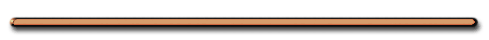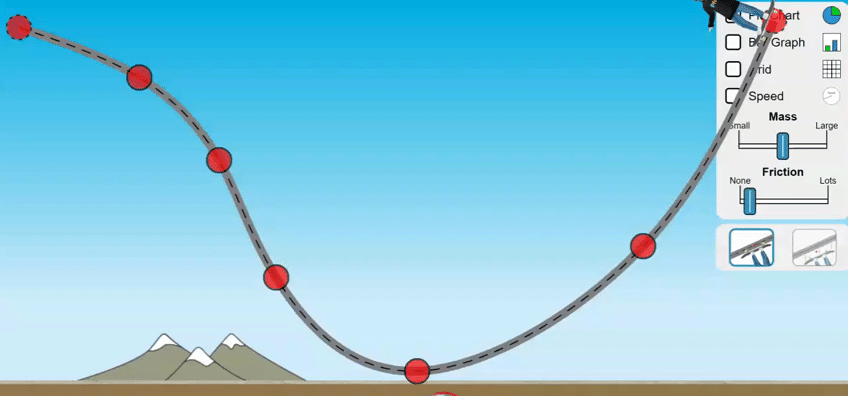Is the Acceleration
Constant, Increasing or Zero?Review

Click on the button and play ...

 Your browser does not support inline frames or is currently configured not to display inline frames. One of these things is not like the other!The idea for this game and graphic come from a great high school physics teacher named Kelly O'Shea.

Try this at home!

Mindpaint Channel

Video Instruction

showmethephysics.com

Fill in the Blanks Notes

IV. Motion Equations for Problem Solving

When an object is moving at a constant acceleration we can use the following equations to find motion informationA. Constant Accel. Equations

(see Reference Table)

1. Average Velocity

V = (Vi + Vf)/2

or

V = Δd/Δt

2. Acceleration:

a = (Vf - Vi)/t

Cross multiply & Solve

at = (Vf - Vi)

 Vf = Vi + at3. Displacement:

V = Δd/Δt

Take Equation 3 and solve for d

Δd = V Δt

• Substitute Equation 1 into previous equation

Δd =[(Vi + Vf)/2]Δt

Since Vf = Vi + at

Δd =[(Vi + (Vi + at)/2]Δt

Gather like terms and solve for d

 Δd =Vit +1/2at2